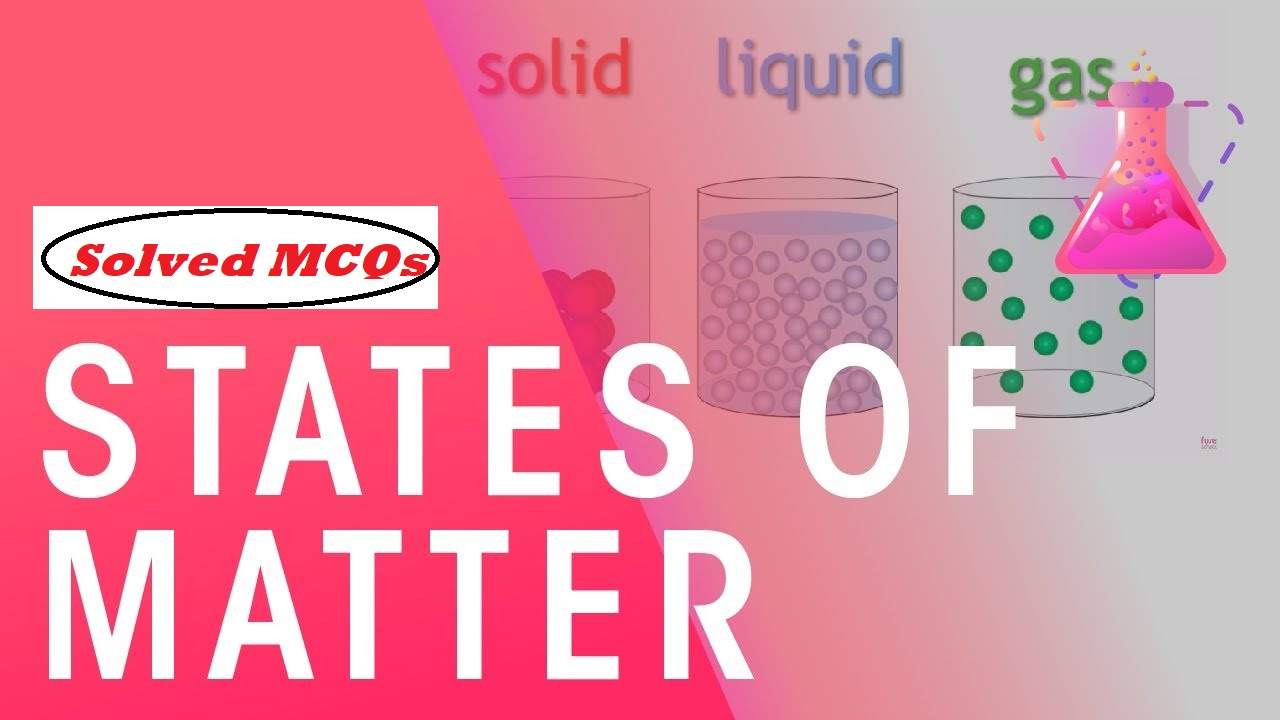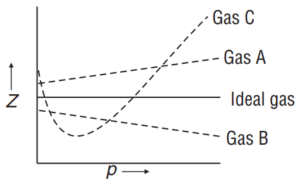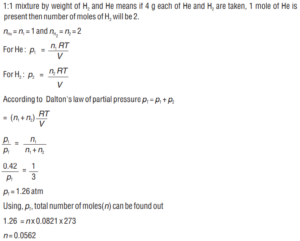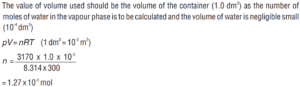# Solved MCQs States of MatterSolved MCQs States of Matter

All questions of Solved MCQs States of Matter are asked in various competitive examinations.

Q1. A sample of gas occupies 100 mL at 27 0C and 740 mm pressure. When its volume is changed to 80 mL at 740 mm pressure, the temperature of the gas will be
(a) 21.6 0C                               (b) 240 0C
(c) – 33 0C                               (d) 89.5 0C
Ans 1. (c) – 33 0C
Since, Pressure is same
According to Charles law
V1/T1 = V2/T2 (T1 = 27 + 273 = 300 K)
100/300 = 80/T2
T2 = 240 K = 240 – 273 = –33 0C
Q2. Which of the following is not the postulate of the kinetic theory of gases?
(a) Gas molecules are in a permanent state of random motion.
(b) Pressure of gas is due to molecular impacts on the walls.
(c) The molecules are perfectly elastic.
(d) The molecular collisions are elastic.
Ans 2. (c) The molecules are perfectly elastic.
Q3. The behavior of real gases approach ideal behavior at
(a) low temperature, low pressure
(b) high temperature, high pressure
(c) low temperature, high pressure
(d) high temperature, low pressure
Ans 3. (d) high temperature, low pressure
Q4. The pressure of a mixture of 4 g of O2 and 2 g of H2 confined in a bulb of 1 litre at 0C is
(a) 25.215 atm                                     (b) 31.205 atm
(c) 45.215 atm                                     (d) 15.210 atm
Ans 4. (a) 25.215 atm
According to the Dalton’s law of partial pressures

pT = p1 + p2
p1 = Partial pressure of O2
p2 = Partial Pressure of H2
pT = Pressure of the mixture or total pressure
p1 = (n1 + n2 )RT/V
on putting the values we get
pT = 25.215 atm

Solved MCQs States of Matter

Q5. Positive deviation from ideal gas behavior takes places because of
(a) Molecular interaction between atoms and pV/nRT> 1
(b) Molecular interaction between atoms and pV/nRT< 1
(c) Finite-size of the atoms and pV/nRT> 1
(d) Finite-size of the atoms and pV/nRT< 1
Ans 5. (a) Molecular interaction between atoms and pV/nRT> 1
Q6. Consider the following plotWhich of the following statement is wrong?
(a) For a gas A, a = 0, and Zwill linearly depend on pressure.
(b) For gas B, b = 0 and Zwill linearly depend on pressure.
(c) Gas C is a real gas and we can find ‘a’ and ‘b’ if intersection data is given.
(d) All van der Waals gases will behave like gas C and give a positive slope at high pressure.
Ans 6. (c) Gas C is a real gas and we can find ‘a’ and ‘b’ if intersection data is given.

Q7. A 1:1 mixture (by weight) of hydrogen and helium is enclosed in a one litre flask kept at 0C. Assuming ideal behavior, the partial pressure of helium is found to be 0.42 atm. Then the concentration of hydrogen would be
(a) 0.0375 M                                       (b) 0.028 M
(c) 0.0562 M                                       (d) 0.0187 M
Ans 7. (a) 0.0375 MNumber of moles of H = n = 2/3 x 0.0562 = 0.0375 mol
Volume = 1 L
Therefore, concentration of H2 = 0.0375 M

Solved MCQs States of Matter

Q8. Critical temperatures of H2O, NH3, CO2, and O2 are 647 K, 405.6 K, 304.10 K, and 154.2 K respectively. If the cooling starts from 500 K to their critical temperature, the gas that liquefies first is
(a) H2O                                                           (b) NH3
(c) CO2                                                            (d) O2
Ans 8. (a) H2O

Q9. The compressibility factor for a real gas at high pressure is
(a) 1                                                     (b) 1 + pb/RT
(c) 1 – pb/RT                                      (d) 1 + RT/pb
Ans 9. (b) 1 + pb/RT

Q10. ‘a’ and ‘b’ are van der Waals constants for gases. Chlorine is more easily liquefied than ethane because
(a) a and b for Cl2 > a and b for C2H6
(b) a and b for Cl2 < a and b for C2H6
(c) a for Cl2 < a for C2H6 but b for Cl2 > b for C2H6
(d) a for Cl2 > a for C2H6 but b for Cl2 < b for C2H6
Ans 10. (d) a for Cl2 > a for C2H6 but b for Cl2 < b for C2H6

Q11. The compressibility of a gas is less than unity at NTP.
(a) Vm > 22.4 L                                               (b) Vm < 22.4 L
(c) Vm = 22.4 L                                               (d) Vm = 44.8 L
Ans 11. (b) Vm < 22.4 L

Q12. Which of the following property of water be used to explain the spherical shape of rain droplets?
(a) viscosity                                                       (b) surface phenomenon
(c) critical phenomena                                    (d) vapour pressure
Ans 12. (b) surface phenomenon

Solved MCQs States of Matter

Q13. The critical temperature of a gas is that temperature:
(a) above which it can no longer remain in the gaseous state
(b) above which it cannot be liquefied by pressure
(c) at which it solidifies
(d) at which the volume of the gas becomes zero
Ans 13. (b) above which it cannot be liquefied by pressure
Q14. If 10 dm3 of water is introduced into a 1.0 dm3 flask at 300 K, how many moles of water are in the vapor phase when equilibrium is established (Given: Vapour pressure of H2O at 300 K is 3170 Pa; R = 8.314 J K-1 mol-1).
(a) 1.27 x 10-3 mol                              (b) 5.56 x 10-3 mol
(c) 1.53 x 10-3 mol                              (d) 4.46 x 10-3 mol
Ans 14. (a) 1.27 x 10-3 molQ15. The molecular velocity of any gas is:
(a) directly proportional to the square of temperature
(b) directly proportional to the square root of temperature
(c) inversely proportional to the square root of temperature
(d) inversely proportional to absolute temperature
Ans 15. (b) directly proportional to the square root of temperature.

Solved MCQs States of Matter